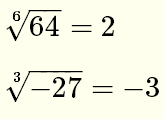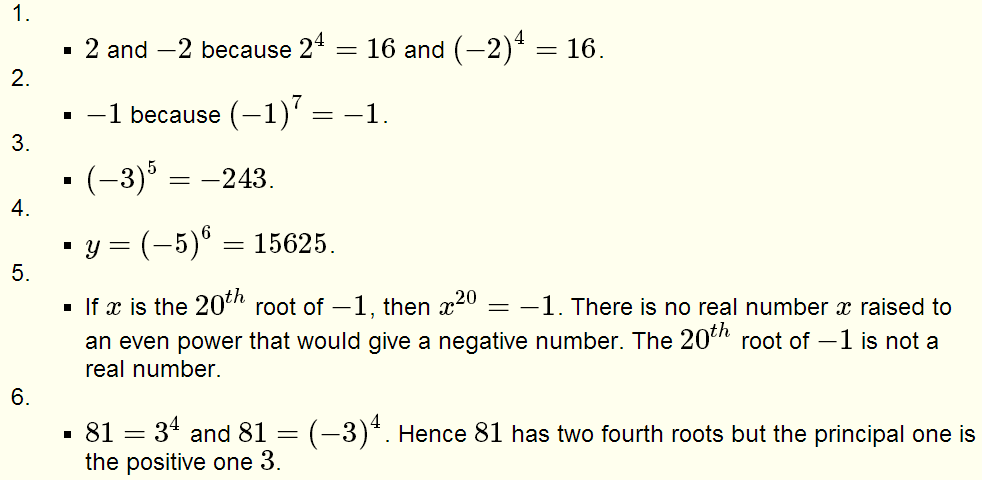# Roots of Real Numbers and Radicals Questions with Solutions

Grade 10 questions on roots of numbers and radicals with solutions are presented.

## Definition

x is the nth root of a number y is equivalent to xn = y.
For
n = 2, the nth root is called the square root.
For
n = 3, the nth root is called the cubic root.

### Examples

1) Since 32 = 9, 3 is the square (n=2) root of 9.
2) Also since
(-3)2 = 9, -3 is also a square root of 9.
3) Since
(-2)3 = -8, -2 is the cubic (n = 3) root of -8
4) Since
34 = 81 and (-3)4 =81, the fourth roots of 81 are 3 and - 3

## Properties of Roots of Real Numbers

1) For n even and y positive, there are two nth roots of y

### Examples

Since 104=10000 and (-10)4 = 10000, the fourth roots of 10000 are 10 and -10.
2) For n even and y negative, there are no real nth roots of y.

### Examples

The square root of - 4 is not a real number since no real number x exists such that x2 = -4
The fourth root of - 16 is not a real number since no real number x exists such that x
4 = -16
3) For n odd, there is always one nth root of y.

### Examples

The cubic (n=3) root of 8 is equal to 2.
The fifth root (n=5) of -100000 is equal to -10

## Principal Root

For n even, the principal root is the positive root. For n odd there is only one root and it is the principal root.

### Examples

The principal 6th of 64 is equal to 2.
The principal cubic root of -64 is equal to - 4.

The symbol √ is called a radical and is used to indicate the principal root of a number as follows:
n√y

where n is called the index of the radical and y is called the radicand.

### ExamplesBecause of its widespread use, the square root of y is written as √y without indicating the index.18. Use a calculator to approximate the following to 3 decimal places:Solutions to the Above Problems